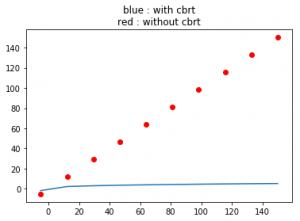# numpy.cbrt() in Python

numpy.cbrt(arr, out = None, ufunc ‘cbrt’) : This mathematical function helps user to calculate cube root of x for all x being the array elements.

Parameters :

```arr  : [array_like] Input array or object
whose elements, we need to square.
```

Return :

```An array with cube root of x for all x i.e. array elements
```

Code #1 : Working

 `# Python program explaining ` `# cbrt () function ` `  `  `import` `numpy as np ` `  `  `arr1 ``=` `[``1``, ``27000``, ``64``, ``-``1000``] ` `print` `(``"cbrt Value of arr1 : \n"``, np.cbrt(arr1)) ` `  `  `arr2 ``=` `[``1024` `,``-``128``] ` `print` `(``"\ncbrt Value of arr2 : "``, np.cbrt(arr2)) `

Output :

```cbrt Value of arr1 :
[  1.  30.   4. -10.]

cbrt Value of arr2 :  [ 10.0793684  -5.0396842]
```

Code #2 : Working with complex numbers

 `# Python program explaining ` `# cbrt () function ` `  `  `import` `numpy as np ` `  `  `a ``=` `4` `+` `3j` `print``(``"cbrt(4 + 3j) : "``, np.cbrt(a)) ` `  `  `b ``=` `16` `+` `13j` `print``(``"\ncbrt value(16 + 13j) : "``, np.cbrt(b)) `

Output :

```TypeError: ufunc 'cbrt' not supported for the input types,
and the inputs could not be safely coerced to any supported
types according to the casting rule ''safe''```

Code #3 : Graphical representation

 `# Python program explaining ` `# cbrt () function ` `  `  `import` `numpy as np ` `import` `matplotlib.pyplot as plt ` `  `  `a ``=` `np.linspace(start ``=` `-``5``, stop ``=` `150``, ` `                ``num ``=` `10``, endpoint ``=` `True``) ` `                  `  `print``(``"Graphical Representation : \n"``, np.cbrt(a)) ` `  `  `plt.title(``"blue : with cbrt\nred : without cbrt"``) ` `plt.plot(a, np.cbrt(a)) ` `  `  `plt.scatter(a, a, color ``=` `'red'``) ` `plt.show() `

Output :

```Graphical Representation :
[-1.70997595  2.30347441  3.08793243  3.60027433  3.99768384  4.3287262
4.61565763  4.87076238  5.10162421  5.31329285]```My Personal Notes arrow_drop_upIf you like GeeksforGeeks and would like to contribute, you can also write an article using contribute.geeksforgeeks.org or mail your article to contribute@geeksforgeeks.org. See your article appearing on the GeeksforGeeks main page and help other Geeks.

Please Improve this article if you find anything incorrect by clicking on the "Improve Article" button below.

Article Tags :

Be the First to upvote.

Please write to us at contribute@geeksforgeeks.org to report any issue with the above content.